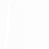## fullrun_ccd0_acisf12156_pi14-548_cl_pass1_csvfiles

#### Source 1

Data taken from csv/fullrun_ccd0_acisf12156_pi14-548_cl_pass1_tfrozen_src1.csv
X132502.69-430243.0
C/dof = 47.04/249
Total counts = 61
Model total counts = 59.6
1 const = 0.01121 (<0.03464)
Model 1
Model 1
2 linx = 0.001883 (-0.00346 - 0.01165)
3 liny = -0.003916 (-0.01858 - 0.004462)
Model 2
Model 2
4 xc = 0.3815 (0.1992 - 0.5564)
5 yc = 0.5356 (0.3619 - 0.7066)
6 norm = 5.892 (4.475 - 7.907)
7 sigmax = 0.6108 (0.542 - 0.6841)
8 sigmay = 0.6108 (= 7)
9 theta = 0 (frozen)
Model 3
Model 3
Model 3 Position (J2000) = 13 25 2.69 -43 2 43.0 (201.261227, -43.045275)
Model 3 Position (xc, yc) = (254.775435, 980.911451)data model resid

#### Source 2

Data taken from csv/fullrun_ccd0_acisf12156_pi14-548_cl_pass1_tfrozen_src2.csv
X132457.03-430934.7
C/dof = 200.45/2296
Total counts = 17
Model total counts = 18.7
Warning, poor agreement between model and actual number of counts may be indicative of a poor fit
1 const = 0.00774 (0.004192 - 0.01191)
Model 1
Model 1
2 linx = -0.0002183 (-0.0008524 - 0.0005696)
3 liny = -5.601e-06 (-0.0008537 - 0.0007379)
Model 2
Model 2
4 xc = 12.08 (>11.88)
5 yc = -12.08 (indef)
6 norm = 1e-06 (<0.01538)
7 sigmax = 5.814 (>5.364)
8 sigmay = 5.814 (= 7)
9 theta = 0 (frozen)
Model 3
Model 3
Model 3 Position (J2000) = 13 24 57.03 -43 9 34.7 (201.237637, -43.159630)
Model 3 Position (xc, yc) = (380.050203, 144.050203)data model resid

#### Source 3

Data taken from csv/fullrun_ccd0_acisf12156_pi14-548_cl_pass1_tfrozen_src3.csv
X132456.70-430813.9
C/dof = 194.05/569
Total counts = 28
Model total counts = 28.0
1 const = 0.01024 (0.00256 - 0.028)
Model 1
Model 1
2 linx = -0.0004951 (-0.00396 - 0.002721)
3 liny = -0.001165 (-0.003456 - 0.001572)
Model 2
Model 2
4 xc = 0.2651 (-0.6485 - 1.165)
5 yc = 0.3455 (-0.6354 - 1.342)
6 norm = 0.2646 (0.1479 - 0.4909)
7 sigmax = 1.799 (1.317 - 2.829)
8 sigmay = 1.799 (= 7)
9 theta = 0 (frozen)
Model 3
Model 3
Model 3 Position (J2000) = 13 24 56.70 -43 8 13.9 (201.236266, -43.137182)
Model 3 Position (xc, yc) = (387.538907, 308.297793)data model resid

#### Source 4

Data taken from csv/fullrun_ccd0_acisf12156_pi14-548_cl_pass1_tfrozen_src4.csv
X132507.43-430409.4
C/dof = -1197.13/249
Total counts = 373
Model total counts = 366.5
1 const = 0.05975 (0.0239 - 0.1119)
Model 1
Model 1
2 linx = 0.01769 (-0.0004248 - 0.03526)
3 liny = -0.02017 (-0.04544 - -0.001139)
Model 2
Model 2
4 xc = 0.03868 (-0.02608 - 0.1055)
5 yc = 0.2926 (0.2266 - 0.3567)
6 norm = 42.6 (37.18 - 48.37)
7 sigmax = 0.5655 (0.5264 - 0.5997)
8 sigmay = 0.5655 (= 7)
9 theta = 0 (frozen)
Model 3
Model 3
Model 3 Position (J2000) = 13 25 7.43 -43 4 9.4 (201.280976, -43.069270)
Model 3 Position (xc, yc) = (149.078625, 805.405232)data model resid